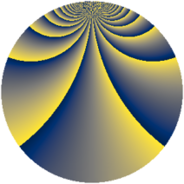# Properties

 Label 1815.2.bdLevel $1815$ Weight $2$ Character orbit 1815.bd Rep. character $\chi_{1815}(164,\cdot)$ Character field $\Q(\zeta_{22})$ Dimension $2600$ Sturm bound $528$

# Related objects

## Defining parameters

 Level: $$N$$ $$=$$ $$1815 = 3 \cdot 5 \cdot 11^{2}$$ Weight: $$k$$ $$=$$ $$2$$ Character orbit: $$[\chi]$$ $$=$$ 1815.bd (of order $$22$$ and degree $$10$$) Character conductor: $$\operatorname{cond}(\chi)$$ $$=$$ $$1815$$ Character field: $$\Q(\zeta_{22})$$ Sturm bound: $$528$$

## Dimensions

The following table gives the dimensions of various subspaces of $$M_{2}(1815, [\chi])$$.

Total New Old
Modular forms 2680 2680 0
Cusp forms 2600 2600 0
Eisenstein series 80 80 0

## Trace form

 $$2600q + 220q^{4} - 66q^{6} - 36q^{9} + O(q^{10})$$ $$2600q + 220q^{4} - 66q^{6} - 36q^{9} - 44q^{10} - 36q^{15} - 284q^{16} - 44q^{19} + 44q^{21} - 42q^{25} + 22q^{30} - 36q^{31} - 60q^{34} - 130q^{36} + 44q^{39} - 2q^{45} - 44q^{46} - 316q^{49} - 44q^{51} + 88q^{54} + 60q^{55} - 4q^{60} - 44q^{61} + 156q^{64} + 92q^{66} - 88q^{69} - 14q^{70} - 8q^{75} - 44q^{76} - 88q^{79} - 20q^{81} + 44q^{84} - 22q^{85} - 110q^{90} - 64q^{91} - 88q^{96} + 106q^{99} + O(q^{100})$$

## Decomposition of $$S_{2}^{\mathrm{new}}(1815, [\chi])$$ into newform subspaces

The newforms in this space have not yet been added to the LMFDB.